# SAS/ETS Web Examples

## Computing Marginal Effects for Discrete Dependent Variable Models

Contents | SAS Program

# Overview

Marginal effects measure the expected instantaneous change in the dependent variable as a function of a change in a certain explanatory variable while keeping all the other covariates constant. The marginal effect measurement is required to interpret the effect of the regressors on the dependent variable. This example illustrates the calculation of marginal effects by using the QLIM procedure in binary choice models and censored models.

# Binary Choice Model

The first data are the binary choice (0–1) data set used by Spector and Mazzeo (1980) to study the effectiveness of a new method in teaching Economics. The data are read by the following DATA step statements.

   data greenedata;
input gpa 1-4 tuce 6-7 psi  grade;
datalines;
2.66 20	0 0
2.89 22	0 0

... more lines ...

3.1  21 1 0
2.39 19 1 1
;
run;


The dependent variableis modeled as follows: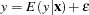where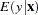is the conditional mean function,is the vector of explanatory variables, andis the error term. The conditional mean function is given by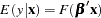wheredenotes a cumulative distribution function and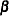denotes the parameters. Therefore,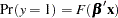Marginal effect is a measure of the instantaneous effect that a change in a particular explanatory variable has on the predicted probability of, when the other covariates are kept fixed. They are obtained by computing the derivative of the conditional mean function with respect togiven by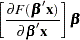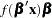where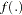is the density function that corresponds to the cumulative function. The marginal effects are nonlinear functions of the parameter estimates and levels of the explanatory variables. Hence, they generally cannot be inferred directly from parameter estimates. Marginal effects for distributions such as probit and logit can be computed with PROC QLIM by using the MARGINAL option in the OUTPUT statement. The following MODEL statement fits the model equation to the endogenous variable GRADE and the covariates GPA, TUCE, and PSI. Further, you can specify the discrete nature of the endogenous variable by using the DISCRETE option. The D=PROBIT option in the MODEL statement enables you to specify the probit distribution. In the OUTPUT statement, use the OUT = option coupled with the MARGINAL option to obtain the marginal effects in the data set.

   proc qlim data=greenedata;
model grade = gpa tuce psi / discrete(d=probit);
output out=outme marginal;
run;
quit;


The following output in Figure 2.1 displays the probit model fit statistics.

Figure 2.1 Parameter Estimates
The QLIM Procedure

Parameter Estimates
Parameter DF Estimate Standard Error t Value Approx
Pr > |t|
Intercept 1 -7.452320 2.542536 -2.93 0.0034
gpa 1 1.625810 0.693869 2.34 0.0191
tuce 1 0.051729 0.083891 0.62 0.5375
psi 1 1.426332 0.595036 2.40 0.0165

The MARGINAL option in PROC QLIM evaluates marginal effects for each observation. In the output data set, OUTME, 'Meff_P2_covariate' and 'Meff_P1_covariate' refer to the marginal effect of 'covariate' on the probability of GRADE=1 and on the probability of GRADE=0, respectively. Hence, 'Meff_P2_gpa' is the marginal effect of GPA on the probability of GRADE=1.

To evaluate the "average" or "overall" marginal effect, two approaches are frequently used. One approach is to compute the marginal effect at the sample means of the data. The other approach is to compute marginal effect at each observation and then to calculate the sample average of individual marginal effects to obtain the overall marginal effect. For large sample sizes, both the approaches yield similar results. However for smaller samples, averaging the individual marginal effects is preferred (Greene 1997, p. 876). This example uses PROC QLIM to compute the overall marginal effect by these two approaches. PROC QLIM outputs the marginal effects computed at each observation in the data set. Hence, in order to obtain overall marginal effect, you can use PROC MEANS to obtain the sample average of individual marginal effects:

   proc means data=outme n mean;
var Meff_P2_gpa Meff_P2_tuce Meff_P2_psi;
title 'Average of the Individual Marginal Effects';
run;
quit;


Figure 2.2 shows the overall marginal effects of PSI, TUCE, and GPA for the probit model fit.

Figure 2.2 Average of Individual Marginal Effects
 Average of the Individual Marginal Effects

The MEANS Procedure

Variable Label N Mean
Meff_P2_gpa
Meff_P2_tuce
Meff_P2_psi
Marginal effect of gpa on the probability of grade=2
Marginal effect of tuce on the probability of grade=2
Marginal effect of psi on the probability of grade=2
 32 32 32
 0.360786 0.0114793 0.31652

Marginal effects at sample means of covariates can be obtained by adding an observation with a missing value for GRADE (endogenous variable) and the sample means of the covariates to the original dataset GREENEDATA. The following DATA step statements add the observation to the original dataset where means of GPA, TUCE, and PSI are 3.117, 21.938, and 0.4375, respectively. Because of the missing value of GRADE, model fitting does not depend on this observation. However, marginal effects and predicted probability values are obtained.

   data me_mean;
datalines;
3.117 21.938 .4375 .
;
run;
data me_mean;
set greenedata me_mean;
run;


Figure 2.3 shows the results of the following statements, which use the MARGINAL option in PROC QLIM on the new data set ME_MEAN:

   proc qlim data=me_mean;
model grade = gpa tuce psi / discrete(d=probit);
output out=outme1 marginal;
run;
quit;


Figure 2.3 Marginal Effects at Covariate Means
 Average of the Individual Marginal Effects

Obs gpa tuce psi grade Meff_P1_gpa Meff_P2_gpa Meff_P1_tuce Meff_P2_tuce Meff_P1_psi Meff_P2_psi
33 3.117 21.938 0.4375 . -0.53325 0.53325 -0.016967 0.016967 -0.46783 0.46783

There might be cases where some regressors are dummy variables. Since the MARGINAL option in PROC QLIM uses derivative calculation, marginal effect results might not be meaningful. In some cases, it might only help to provide a reasonable approximation. In such cases, analyzing the marginal effects due to dummy variables by taking the difference of estimated probabilities between the different levels of dummy covariates (PSI=1 and PSI=0) is more appropriate. Letdenote the dummy explanatory variable and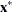denote the other covariates at their means. The effect due to a change ofon the predicted probabilities ofis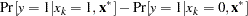The PROBALL option in the OUTPUT statement enables you to obtain the predicted probabilities of discrete endogenous variables for all responses. In order to obtain the previously described difference (when the other covariates are fixed at their means), you can append two observations with 1 and 0 as the discrete regressor’s (PSI) value, means of the other covariates, and missing values for the response variable as shown.

   data me_diff;
datalines;
3.117 21.938 1 .
3.117 21.938 0 .
;
run;

   data me_diff;
set greenedata me_diff;
run;
proc qlim data=me_diff;
model grade = gpa tuce psi / discrete(d=probit);
output out=outme2 marginal proball;
run;
quit;


The marginal effect of PSI is computed as follows: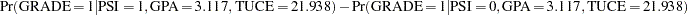The table obtained by using the PROBALL option in PROC QLIM produces two columns labeled 'Prob2_grade' and 'Prob1_grade' which areand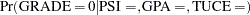respectively. You can obtain the predicted probabilities required for the above computation of marginal effects from the last two observations in column 'Prob2_grade'. As shown in Figure 2.4 , you can compute the marginal effect of PSI to be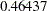(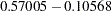). Hence, the overall effect of a unit change in PSI (by introducing the new teaching method with an exposure to PSI), on the probability of GRADE=1 (an increase in the student’s grade) is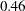.

Figure 2.4 Estimated Probability by Using the PROBALL Option
 Average of the Individual Marginal Effects

33 3.117 21.938 1 . -0.63858 0.63858 -0.020318 0.020318 -0.56023 0.56023 0.42995 0.57005
34 3.117 21.938 0 . -0.29701 0.29701 -0.009450 0.009450 -0.26057 0.26057 0.89432 0.10568

Note that although for discrete covariates the difference calculation is usually employed to obtain marginal effects, in this example you can observe that both the derivative (Meff_P2_psi=, from Figure 2.3) and difference calculations (, by taking the difference of probabilities under 'Prob2_grade' from Figure 2.4) produce similar results. However, this might not be true for other data sets. The marginal effects plot with respect to PSI on theis shown in Figure 2.5 using results from the probit model fit. The marginal effects of PSI onare obtained as a function of the GPA, at the mean of TUCE. This allows better interpretation of results. As shown in Figure 2.5, you can conclude that the effect of PSI is much greater on those students who have a higher GPA.

Figure 2.5 Marginal Effect of PSI on Predicted Probabilities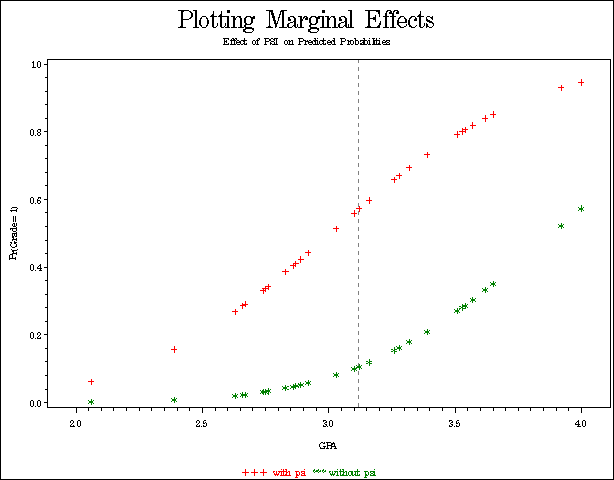Similar analysis with ordered multinomial, censored, and truncated data are supported by the QLIM procedure. An example with censored data is illustrated below.

# Censored Regression Model

In this section an illustration of the marginal effect calculation for censored regression model is given. The censored regression model is described as follows: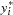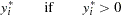where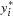is the latent variable and
is the observed variable. The marginal effect in this case is as follows: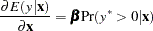The Mroz (1987) data set taken from the 1976 panel study of income dynamics based on data obtained for the previous year 1975, is used for analysis. The dataset is used in the study of female labor supply. A tobit model is fitted to the data. The following variables are used in the model:

• WHRS = wife’s hours of work in 1975

• LFP = dummy variable; LFP = 1 if woman worked in 1975; else = 0

• KL6 = number of children less than 6 years old in household

• K618 = number of children between ages 6 and 18 in household

• WA = wife’s age

• WE = wife’s educational attainment, in years

• HHRS = husband’s hours worked in 1975

• HA = husband’s age

• HE = husband’s educational attainment, in years

• HW = husband’s wage, in 1975 dollars

• FAMINC = family income, in 1975 dollars

The following DATA step statements are used to read the data.

   data mroz;
infile datalines truncover;
input LFP WHRS KL6 K618 WA WE WW RPWG HHRS HA HE HW FAMINC MTR WMED WFED UN CIT AX;
datalines;
1 1610 1 0 32 12  3.3540  2.65 2708 34 12  4.0288 16310 .7215 12  7  5.0 0 14
1 1656 0 2 30 12  1.3889  2.65 2310 30  9  8.4416 21800 .6615  7  7 11.0 1  5
1 1980 1 3 35 12  4.5455  4.04 3072 40 12  3.5807 21040 .6915 12  7  5.0 0 15

... more lines ...



In this example, a tobit model is estimated. You can fit a logit or a probit model to the dummy response variable LFP, similar to the analysis shown earlier. You can append the MROZ data set with the mean values of the explanatory variables as shown in the following statements.

   /*last row has the means*/
input LFP WHRS KL6 K618 WA WE HHRS HA HE HW FAMINC ;
datalines;
1 740.5763612 0.237715803 1.353253652 42.53784861 12.28685259 2267.270916 45.12084993 12.49136786 7.482178752 23080.59495
;
run;

   data mrozexsub;
set mroz(keep=LFP WHRS KL6 K618 WA WE HHRS HA HE HW FAMINC) add;
run;


Figure 2.6 shows the marginal effect results for a tobit model fit.


proc qlim data=mrozexsub;
model WHRS = KL6 K618 WA WE HHRS HA HE HW FAMINC;
endogenous WHRS ~ censored(lb=0);
output out=outtobit residual marginal;
run;

   proc print data=outtobit(firstobs=754);
var Meff_KL6 Meff_K618 Meff_WA Meff_WE Meff_HHRS Meff_HA Meff_HE Meff_HW Meff_FAMINC;
run;


Figure 2.6 Marginal Effects for a Tobit Model Fit
Obs Meff_KL6 Meff_K618 Meff_WA Meff_WE Meff_HHRS Meff_HA Meff_HE Meff_HW Meff_FAMINC
754 -579.163 -63.9936 -17.2332 52.7183 -0.43955 -10.7785 -3.79460 -127.807 0.039525

Similar analysis using the probit and logit models yield results shown in Figure 2.7 and Figure 2.8 respectively.

Figure 2.7 Marginal Effects for a Probit Model Fit
Obs Meff_P1_KL6 Meff_P1_K618 Meff_P1_WA Meff_P1_WE Meff_P1_HHRS Meff_P1_HA Meff_P1_HE Meff_P1_HW Meff_P1_FAMINC
754 0.34706 0.020687 0.011396 -0.056156 .000255579 .007300417 .006522166 0.068965 -.000021673

Figure 2.8 Marginal Effects for a Logit Model Fit
Obs Meff_P1_KL6 Meff_P1_K618 Meff_P1_WA Meff_P1_WE Meff_P1_HHRS Meff_P1_HA Meff_P1_HE Meff_P1_HW Meff_P1_FAMINC
754 0.35548 0.019558 0.011089 -0.058672 .000274938 .008184995 .006452848 0.074250 -.000023536

# References

Greene, W. H. (1997), Econometric Analysis, Third edition, Prentice Hall, 339–350.

Mroz, T.A. (1987), "The Sensitivity of an Empirical Model of Married Women’s Hours of Work to Economic and Statistical Assumptions," Econometrica, 55, 765–799.

Spector, L. and Mazzeo, M. (1980), "Probit Analysis and Economic Education," Journal of Economic Education, 11, 37–44.

SAS Institute Inc. (2008), SAS/ETS User’s Guide, Version 9.2, Cary, NC: SAS Institute Inc.